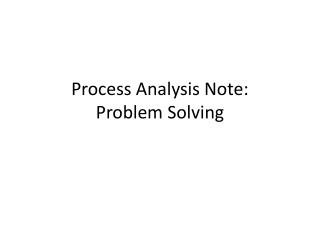DownloadDownload PresentationProcess Analysis Note: Problem Solving

# Process Analysis Note: Problem Solving

Download Presentation## Process Analysis Note: Problem Solving

- - - - - - - - - - - - - - - - - - - - - - - - - - - E N D - - - - - - - - - - - - - - - - - - - - - - - - - - -
##### Presentation Transcript

1. Process Analysis Note:Problem Solving

2. 1. The copy center has a job order that requires 500 pages of white and 200 pages of pink. The copying rate is 25 pages per minute, the overall setup time is 5 minutes, and the color change time is 2 minutes. What is the expected capacity requirement for the copy machine center to do this job? • Answer: set-up = 5 minutes run = (500 + 200)/25 = 28 minutes color change =2 minutes 35 minutes

3. 2. The routing for the job in Problem 1 also calls for stapling and three-hole drilling (in that order). The time standards are 10 minutes and 20 minutes, respectively. If labor cost is charged on the basis of total standard time multiplied by \$6.00 per hour, estimate the cost of this job, including the work in Problem 1. Answer: 35 + 10 + 20 = 65 min/job x 1 hour/60 min = 1.083 hours x \$6.00 /hour = \$6.50/job

4. 3. If the job has to wait in queue at the copy work center for 24 minutes, at the stapling work center for 43 minutes, and at the three-hole drilling work center for 17 minutes, what is the throughput time for this job? Answer: 24 + 35 + 43 + 10 + 17 + 20 = 149 mi But this turns out to be a tricky question. : If all jobs were copy-staple-drill with the tasks times as given, these waits would not occur. Waits would occur prior to the bottleneck task.

5. 4. Suppose the copy center only did jobs exactly like this one. What would the cycle time be? Assume that different people work in each of the three work centers. Answer: 35 min.

6. 5. What is the maximum number of such jobs that can be produced in a 40 hour week? Answer: • If it is assumed that the process is in steady state, that jobs can be stopped and restarted at any point, and that the 40 hour week is all available work time (no breaks subtracted), the calculation is: (40 hours/week x 60 minutes/hour)/(35 minutes/job) = 68.6 jobs/week • If it is assumed that the first job starts the operation "from scratch", the time required for the first job is 65 minutes, so the calculation would subtract 65 minutes from the total time in the week and divide by the cycle time, then add back the first job (which was subtracted from the total time) to get: (2400 - 65)/35 +1 = 67.7 jobs/week • If it is assumed that breaks are subtracted from the 40 hour work week, total time available is reduced. • If it is assumed that jobs cannot be stopped in the middle, the calculation is more complicated and depends on when breaks are factored in (it is probably not reasonable to assume that the 8 hours are worked without any breaks at all).

7. 6. What is the capacity utilization of each of the three work centers when the operating unit works at the maximum rate as determined in problem 5? Answer: • Copy = 35/35 = 100% • Staple = 10/35 = 28.6% • Drill = 20/35 = 57.1%

8. 7. How do the answers to problems 1-6 change if the batch size is doubled? Answer: The copy task time becomes 63 minutes (5 + (1400/25) + 2); set-up and run for the task are not affected. Assumptions need to be made regarding the effect of the batch size increase on the staple and drill tasks It is not half capacity!

9. 8. If there are 20 jobs on the average in inventory, and the copy center turns out 50 jobs per month, what is the inventory turnover rate? Answer: 50/20 = 2.5

10. 9. Suppose that in problem 1, 750 sheets of paper were required in order to complete the job. What is the yield? the scrap rate? Answer: Yield = 700/750 = 93.3% Scrap = 1-yield = 6.7%

11. 10. As a customer, what criteria might you use to evaluate the quality of the copy center's product and service? Answer: The quality characteristics of importance include: - On-time delivery - Speed of service - Friendliness of service - Value of packet - Quality of print - Completeness of packet - Comfort of wait in line - Length of wait in line - Completeness of information provided (pre-assignment, required versus suggested readings, etc.) These may have different weights for different customer groups and for different individual customers.